# Modeling a serial dilution experiment on different liquid handling/dispensing devices.¶

A recent paper by Ekins et al. [Dispensing Processes Impact Apparent Biological Activity as Determined by Computational and Statistical Analyses. PLoS ONE 8(5): e62325, 2013], a large discrepany was observed in IC50 assay values in which either a LabCyte Echo acoustic dispensing unit or a Tecan Genesis liquid handling workstation were used in performing the assays. This discrepancy may be due to any number of factors, but one of the main features of the Echo is its direct dispensing technology that allows a dilution series over several orders of magnitude in concetration to be created directly without the need for serial dilution. Could the observed discrepancy be explained by the difference in the accuracy with which a serial dilution can be created by these two technologies? A little modeling of the experiment can quickly find out!

In :
import numpy
from pylab import *


## Dilution and dispensing by a liquid-handling robot¶

Let's model how a liquid-handling robot creates a dilution series for a compound via serial dilution. An ideal dilution series has no error in transferred volumes and ensures complete mixing between dilution steps.

In :
def dilution_series(V0, C0, Vtransfer, Vbuffer, ndilutions):
import numpy
from numpy.random import normal
ideal_concentrations = numpy.zeros([ndilutions], numpy.float64)
ideal_volumes = numpy.zeros([ndilutions], numpy.float64)
ideal_concentrations = C0
ideal_volumes = V0
for n in range(1,ndilutions):
ideal_concentrations[n] = ideal_concentrations[n-1] * Vtransfer / (Vtransfer + Vbuffer)
ideal_volumes[n] = Vtransfer + Vbuffer
ideal_volumes[n-1] -= Vtransfer
ideal_volumes[ndilutions-1] -= Vtransfer
return [ideal_volumes, ideal_concentrations]


Let's consider a 12-point 2x dilution series, where we start with 10 mM compound stocks.

In :
ndilutions = 8 # number of points in dilution series (including original concentration)
V0 = 100e-6 # 100 uL final volume in each well
C0 = 10e-3 # 10 mM initial DMSO stock
Vtransfer = 50e-6 # 50 uL transfer from previous dilution or stock
Vbuffer = 50e-6 # 50 uL buffer
[ideal_volumes, ideal_concentrations] = dilution_series(V0, C0, Vtransfer, Vbuffer, ndilutions)

# Font size settings
font = {'family' : 'sans serif',
'weight' : 'normal',
'size'   : 12}
matplotlib.rc('font', **font)

# Plot ideal concentrations.
clf()
semilogy(range(ndilutions), ideal_concentrations, 'ko');
xlabel('dilution number');
ylabel('concentration (M)');
axis([-0.5, ndilutions, 0.0, C0*1.05]);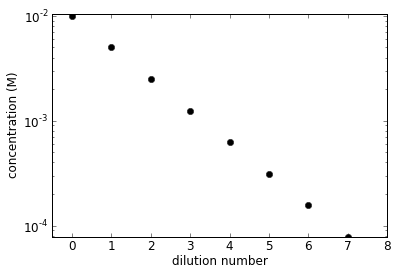Real liquid-handling robots can't transfer the specified volume exactly. Each transfer operation has some inaccuracy (modeled as a constant bias factor for all dispensing operations) and imprecision (random error associated with each volume transfer). We'll ignore other contributions to error, such as compound stickiness, insolubility, etc.

In :
def robot_dilution_series(V0, C0, Vtransfer, Vbuffer, ndilutions, pipetting_model):
[transfer_inaccuracy, transfer_imprecision] = pipetting_model(Vtransfer)
[buffer_inaccuracy, buffer_imprecision] = pipetting_model(Vtransfer)

from numpy.random import normal
actual_concentrations = numpy.zeros([ndilutions], numpy.float64)
actual_volumes = numpy.zeros([ndilutions], numpy.float64)
actual_concentrations = C0
actual_volumes = V0
transfer_bias = transfer_inaccuracy * normal()
buffer_bias = buffer_inaccuracy * normal()
for n in range(1,ndilutions):
Vtransfer_actual = Vtransfer * ((1+transfer_bias) + transfer_imprecision*normal())
Vbuffer_actual = Vbuffer * ((1+buffer_bias) + buffer_imprecision*normal())
actual_concentrations[n] = actual_concentrations[n-1] * Vtransfer_actual / (Vtransfer_actual + Vbuffer_actual)
actual_volumes[n] = Vtransfer_actual + Vbuffer_actual
actual_volumes[n-1] -= Vtransfer_actual
Vtransfer_actual = Vtransfer * ((1+transfer_bias) + transfer_imprecision*normal())
actual_volumes[ndilutions-1] -= Vtransfer_actual
return [actual_volumes, actual_concentrations]


Suppose we generate this dilution series using a Tecan Genesis robot used in the assay.

I couldn't find published specs for standard bias and error for the Genesis (which appears to be no longer produced), so we'll use data for a Beckman NX/FX span-8 instead:

inaccuracy/imprecision data for Biomek NX/FX

In :
def tecan_genesis_pipetting_model(volume):
# Assume published imprecision/inaccuracy for Beckman Biomek NX/FX span-8.
from scipy.interpolate import interp1d
imprecision_function = interp1d([0.5e-6, 1e-6, 5e-6, 10e-6, 50e-6, 100e-6, 250e-6, 950e-6], [0.10, 0.07, 0.05, 0.05, 0.05, 0.05, 0.02, 0.01]) # published imprecision for Beckman NX/FX span-8
inaccuracy_function = interp1d([0.5e-6, 1e-6, 100e-6, 250e-6, 900e-6], [0.05, 0.03, 0.03, 0.02, 0.01]) # published inaccuracy for Beckman NX/FX span-8
return [inaccuracy_function(volume), imprecision_function(volume)]

# Biomek NX or FX specs
[actual_volumes, actual_concentrations] = robot_dilution_series(V0, C0, Vtransfer, Vbuffer, ndilutions, tecan_genesis_pipetting_model)

# Plot ideal and actual concentrations.
clf()
semilogy(range(ndilutions), ideal_concentrations, 'ko', range(ndilutions), actual_concentrations, 'ro');
xlabel('dilution number');
ylabel('concentration (M)');
legend(['ideal','actual']);
axis([-0.5, ndilutions, 0.0, C0*1.05]);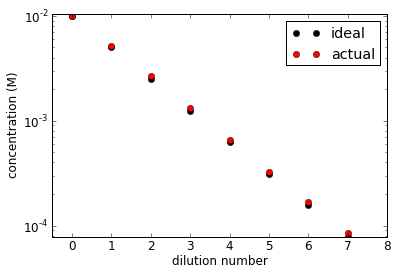Let's plot the relative errors in concentration, volume, and total quantity of compound per well generated in this particular replicate of the dilution series:

In :
clf();
hold(True);
plot([0, ndilutions], [1, 1], 'k-');
plot(range(ndilutions), actual_concentrations / ideal_concentrations, 'ro', range(ndilutions), actual_volumes / ideal_volumes, 'go', range(ndilutions), (actual_volumes*actual_concentrations)/(ideal_volumes*ideal_concentrations), 'bo');
legend(['exact', 'concentration', 'volume', 'quantity'], loc='lower right');
axis([0, ndilutions, 0, 1.5]);
ylabel('relative quantity');
xlabel('dilution number');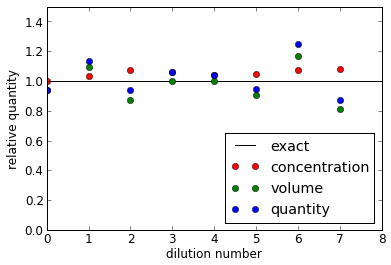If we repeat the experiment many times, we can estimate the CV for each dilution number.

In :
nreplicates = 5000
actual_volumes_n = numpy.zeros([nreplicates, ndilutions], numpy.float64)
actual_concentrations_n = numpy.zeros([nreplicates, ndilutions], numpy.float64)
for replicate in range(nreplicates):
[actual_volumes_replicate, actual_concentrations_replicate] = robot_dilution_series(V0, C0, Vtransfer, Vbuffer, ndilutions, tecan_genesis_pipetting_model)
actual_volumes_n[replicate,:] = actual_volumes_replicate
actual_concentrations_n[replicate,:] = actual_concentrations_replicate

# Compute CVs
volumes_cv = (actual_volumes_n / ideal_volumes).std(0)
concentrations_cv = (actual_concentrations_n / ideal_concentrations).std(0)
quantity_cv = ((actual_volumes_n * actual_concentrations_n) / (ideal_volumes * ideal_concentrations)).std(0)

# Plot CVs
clf()
x = numpy.arange(ndilutions)
plot(x, concentrations_cv*100, 'ro', x, volumes_cv*100, 'go', x, quantity_cv*100, 'bo');
xlabel('dilution number');
ylabel('CV (%)');
legend(['concentration', 'volume', 'quantity'], loc='upper left');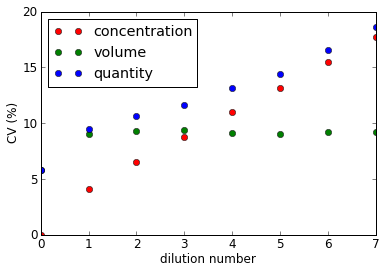The biases may also be important, so let's plot those too.

In :
# Compute relative bias.
volumes_bias = (actual_volumes_n / ideal_volumes).mean(0) - 1
concentrations_bias = (actual_concentrations_n / ideal_concentrations).mean(0) - 1
quantity_bias = ((actual_volumes_n * actual_concentrations_n) / (ideal_volumes * ideal_concentrations)).mean(0)- 1

# Plot relative biases.
clf()
x = numpy.arange(ndilutions)
plot([0, ndilutions], [0, 0], 'k-', x, concentrations_bias*100, 'ro', x, volumes_bias*100, 'go', x, quantity_bias*100, 'bo');
xlabel('dilution number');
ylabel('bias (%)');
legend(['concentration', 'volume', 'quantity'], loc='lower right');
axis([0, ndilutions, -100, 100]);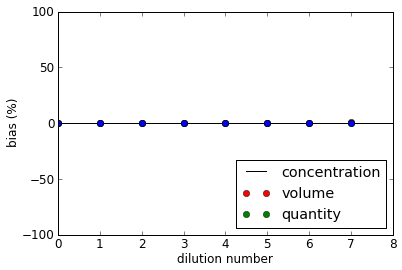In :
def robot_dilution_series(V0, C0, Vtransfer, Vbuffer, ndilutions, pipetting_model):
[transfer_inaccuracy, transfer_imprecision] = pipetting_model(Vtransfer)
[buffer_inaccuracy, buffer_imprecision] = pipetting_model(Vtransfer)

# Account for dilution effect.
from scipy.interpolate import interp1d
x = numpy.array([20, 200]) * 1e-6 # L
y = numpy.array([-0.0630, -0.0496]) # doi:10.1016/j.jala.2006.02.005
dilution_function = interp1d(x, y)

from numpy.random import normal
actual_concentrations = numpy.zeros([ndilutions], numpy.float64)
actual_volumes = numpy.zeros([ndilutions], numpy.float64)
actual_concentrations = C0
actual_volumes = V0
transfer_bias = transfer_inaccuracy * normal()
buffer_bias = buffer_inaccuracy * normal()
for n in range(1,ndilutions):
Vtransfer_actual = Vtransfer * ((1+transfer_bias) + transfer_imprecision*normal())
Vbuffer_actual = Vbuffer * ((1+buffer_bias) + buffer_imprecision*normal())
actual_concentrations[n] = actual_concentrations[n-1] * Vtransfer_actual / (Vtransfer_actual + Vbuffer_actual) * (1+dilution_function(Vtransfer))
actual_volumes[n] = Vtransfer_actual + Vbuffer_actual
actual_volumes[n-1] -= Vtransfer_actual
Vtransfer_actual = Vtransfer * ((1+transfer_bias) + transfer_imprecision*normal())
actual_volumes[ndilutions-1] -= Vtransfer_actual
return [actual_volumes, actual_concentrations]

nreplicates = 5000
actual_volumes_n = numpy.zeros([nreplicates, ndilutions], numpy.float64)
actual_concentrations_n = numpy.zeros([nreplicates, ndilutions], numpy.float64)
for replicate in range(nreplicates):
[actual_volumes_replicate, actual_concentrations_replicate] = robot_dilution_series(V0, C0, Vtransfer, Vbuffer, ndilutions, tecan_genesis_pipetting_model)
actual_volumes_n[replicate,:] = actual_volumes_replicate
actual_concentrations_n[replicate,:] = actual_concentrations_replicate

In :
# Compute CVs
volumes_cv = (actual_volumes_n / ideal_volumes).std(0)
concentrations_cv = (actual_concentrations_n / ideal_concentrations).std(0)
quantity_cv = ((actual_volumes_n * actual_concentrations_n) / (ideal_volumes * ideal_concentrations)).std(0)

# Plot CVs
clf()
x = numpy.arange(ndilutions)
plot(x, concentrations_cv*100, 'ro', x, volumes_cv*100, 'go', x, quantity_cv*100, 'bo');
xlabel('dilution number');
ylabel('CV (%)');
legend(['concentration', 'volume', 'quantity'], loc='lower right');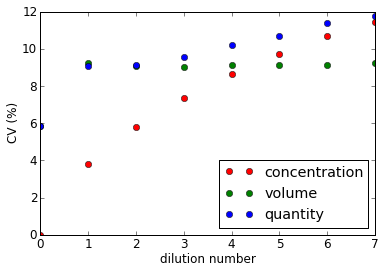In :
# Compute relative bias.
volumes_bias = (actual_volumes_n / ideal_volumes).mean(0) - 1
concentrations_bias = (actual_concentrations_n / ideal_concentrations).mean(0) - 1
quantity_bias = ((actual_volumes_n * actual_concentrations_n) / (ideal_volumes * ideal_concentrations)).mean(0)- 1

# Plot relative biases.
clf()
x = numpy.arange(ndilutions)
plot([0, ndilutions], [0, 0], 'k-', x, concentrations_bias*100, 'ro', x, volumes_bias*100, 'go', x, quantity_bias*100, 'bo');
xlabel('dilution number');
ylabel('bias (%)');
legend(['none', 'concentration', 'volume', 'quantity'], loc='upper right');
axis([0, ndilutions, -100, 100]);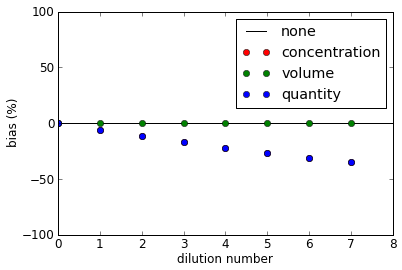If we now dispense 2 uL of diluted compound into an assay plate well into which 10 uL of enzyme mix has been dispensed, there is additional error since the imprecision at this volume is higher.

In :
compound_volume = 2.0e-6 # 2 uL
mix_volume = 10.0e-6 # 10 uL
def robot_dispense(compound_volume, mix_volume, compound_concentrations, pipetting_model):
[compound_inaccuracy, compound_imprecision] = pipetting_model(compound_volume)
[mix_inaccuracy, mix_imprecision] = pipetting_model(mix_volume)

from numpy.random import normal
assay_volume = numpy.zeros([ndilutions], numpy.float64)
assay_compound_concentration = numpy.zeros([ndilutions], numpy.float64)

compound_bias = compound_inaccuracy * normal()
mix_bias = mix_inaccuracy * normal()

for i in range(ndilutions):
compound_volume_dispensed = compound_volume * ((1+compound_bias) + compound_imprecision*normal())
mix_volume_dispensed = mix_volume * ((1+mix_bias) + mix_imprecision*normal())
assay_volume[i] = compound_volume_dispensed + mix_volume_dispensed
assay_compound_concentration[i] = compound_concentrations[i] * compound_volume_dispensed / assay_volume[i]

return [assay_volume, assay_compound_concentration]

[assay_volumes, assay_compound_concentrations] = robot_dispense(compound_volume, mix_volume, actual_concentrations, tecan_genesis_pipetting_model)

pyplot.clf()
pyplot.plot([0,ndilutions], [1, 1], 'k-', range(ndilutions), assay_volumes / (compound_volume+mix_volume), 'ro', range(ndilutions), assay_compound_concentrations / (ideal_concentrations*compound_volume/(compound_volume+mix_volume)), 'go')
pyplot.legend(['exact', 'assay well volume rel err', 'concentration rel err'], loc='lower left')
pyplot.xlabel('dilution number')
pyplot.ylabel('relative error')
pyplot.show()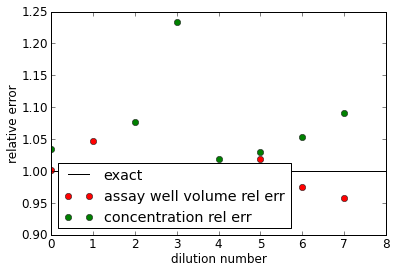Let's suppose the 10 uL of assay mix in each well was dispensed completely accurately, and contains exact concentrations of enzyme and substrate. We presume inhibition is measured by an exact read of the reaction V0/Vmax. The IC50 would be the interpolated point at which V0/Vmax drops to 0.5, as determined from a fit of the competitive inhibition equations to the observed V0/Vmax for the dilution series, using the ideal dilution series concentrations in the fit. Let's assume the true Ki is 10 nM, with Km of 1.71 uM (the Km for ATP by EphB4). Note that we're assuming that EphB4 obeys Michaelis-Menten kinetics here, and that V0/Vmax is what is measured.

In :
def competitive_inhibition(substrate_concentration, inhibitor_concentration, enzyme_concentration, Ki, Km):
V0_over_Vmax = substrate_concentration / (Km*(1 + inhibitor_concentration/Ki) + substrate_concentration)
return V0_over_Vmax

substrate_concentration = 4e-6 # 4 uM ATP
enzyme_concentration = 6e-6 # 0.25 ng of ~42.4 kDa enzyme = ~6 uM
true_Ki = 10e-9 # 10 nM
Km = 1.71e-6 # ATP Km from http://www.proqinase.com/kinase-database/pdfs/2843.pdf

activity = numpy.zeros([ndilutions], numpy.float64)
for i in range(ndilutions):
activity[i] = competitive_inhibition(substrate_concentration, assay_compound_concentrations[i], enzyme_concentration, true_Ki, Km)

pyplot.clf()
pyplot.semilogx(ideal_concentrations, activity, 'ro')
pyplot.xlabel('ideal [I] (M)')
pyplot.ylabel('V0/Vmax')
pyplot.show()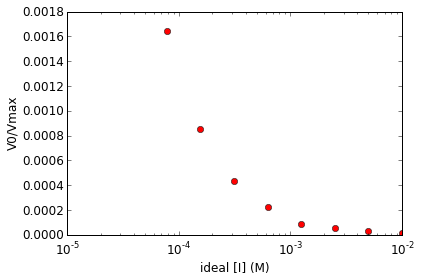What does this look like if we run many assay replicates?

In :
nreplicates = 1000
activity = numpy.zeros([nreplicates,ndilutions], numpy.float64)
for replicate in range(nreplicates):
[actual_volumes, actual_concentrations] = robot_dilution_series(V0, C0, Vtransfer, Vbuffer, ndilutions, tecan_genesis_pipetting_model)
[assay_volumes, assay_compound_concentrations] = robot_dispense(compound_volume, mix_volume, actual_concentrations, tecan_genesis_pipetting_model)
for i in range(ndilutions):
activity[replicate,i] = competitive_inhibition(substrate_concentration, assay_compound_concentrations[i], enzyme_concentration, true_Ki, Km)

pyplot.clf()
pyplot.semilogx(ideal_concentrations, activity.transpose(), 'r-')
pyplot.xlabel('ideal [I] (M)')
pyplot.ylabel('V0/Vmax')
pyplot.show()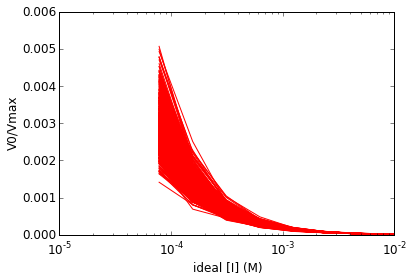It's not hard to see that the IC50 will vary over a large range! Let's fit the IC50s to see.

In :
def fit_ic50(inhibitor_concentrations, activities):
import numpy, scipy
def objective(inhibitor_concentrations, Ki):
activities = numpy.zeros([ndilutions], numpy.float64)
for i in range(ndilutions):
activities[i] = competitive_inhibition(substrate_concentration, inhibitor_concentrations[i], enzyme_concentration, Ki, Km)
return activities

Ki_guess = true_Ki
import scipy.optimize
[popt, pcov] = scipy.optimize.curve_fit(objective, inhibitor_concentrations, activities, p0=[Ki_guess])

return popt

IC50s_tips = numpy.zeros([nreplicates], numpy.float64)
for replicate in range(nreplicates):
IC50s_tips[replicate] = fit_ic50(ideal_concentrations, activity[replicate,:])
pIC50s_tips = numpy.log10(IC50s_tips)

clf()
nhist = 20
hist(pIC50s_tips, nhist);
xlabel('measured pIC50 (M)');
ylabel('P(pIC50)');
gca().axes.get_yaxis().set_ticks([]); # turn off y ticks

Out:
[]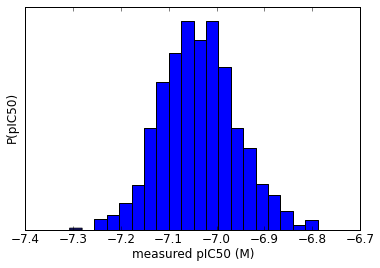The error won't be uniform across the whole Ki range, however. Let's characterize the standard error as a function of Ki.

In :
def robot_IC50s(true_Ki):
nreplicates = 1000
IC50s = numpy.zeros([nreplicates], numpy.float64)
for replicate in range(nreplicates):
[actual_volumes, actual_concentrations] = robot_dilution_series(V0, C0, Vtransfer, Vbuffer, ndilutions, tecan_genesis_pipetting_model)
[assay_volumes, assay_compound_concentrations] = robot_dispense(compound_volume, mix_volume, actual_concentrations, tecan_genesis_pipetting_model)
activities = numpy.zeros([ndilutions], numpy.float64)
for i in range(ndilutions):
activities[i] = competitive_inhibition(substrate_concentration, assay_compound_concentrations[i], enzyme_concentration, true_Ki, Km)
IC50s[replicate] = fit_ic50(ideal_concentrations, activities)
return IC50s

pKis = numpy.array([-12, -11, -10, -9, -8, -7, -6, -5, -4, -3], numpy.float64);
Kis = 10**pKis
nKis = len(pKis)
genesis_pIC50_bias = numpy.zeros([nKis], numpy.float64)
genesis_pIC50_CV = numpy.zeros([nKis], numpy.float64)
for (i, Ki) in enumerate(Kis):
IC50s = robot_IC50s(Ki)
pIC50s = numpy.log10(IC50s)
pKi = pKis[i]
genesis_pIC50_bias[i] = pIC50s.mean() - pKi;
genesis_pIC50_CV[i] = pIC50s.std() / abs(pIC50s.mean())


We can plot the bias in the measured pIC50 values.

In :
clf();
plot([pKis.min(), pKis.max()], [0, 0], 'k-', pKis, genesis_pIC50_bias, 'ro');
xlabel('pKi');
ylabel('bias in measured pIC50');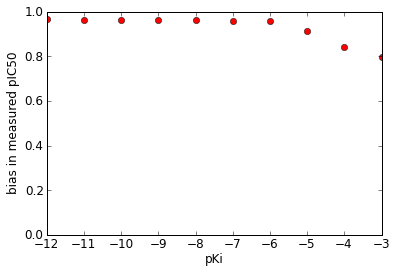The CV is not so bad.

In :
clf();
plot(pKis, genesis_pIC50_CV*100, 'ko');
xlabel('pKi');
ylabel('pIC50 CV (%)');
axis([pKis.min(), pKis.max(), 0, 10]);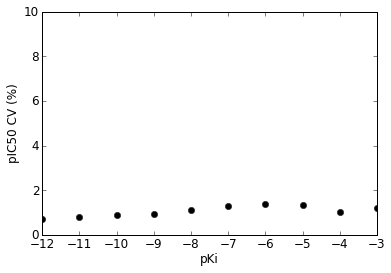## Dispensing by LabCyte Echo¶

What about the LabCyte Echo experiments? According to LabCyte, the Echo has an inaccuracy of 10% and a precision of 8% over the entire dispense range of 2.5 nL to 10 uL. Let's model how it dispenses directly into the assay plate with an 9-point dilution series spanning 2.5 nL to 120 nL from 10 mM stock solution.

In :
def echo_assay_dispense(C0, mix_volume, backfill_volume, ideal_dispense_volumes):
inaccuracy = 0.10 # Specs from: http://www.labcyte.com/sites/default/files/support_docs/Echo%205XX%20Specifications.pdf
imprecision = 0.08 # Specs from: http://www.labcyte.com/sites/default/files/support_docs/Echo%205XX%20Specifications.pdf
from numpy.random import normal
ndilutions = len(ideal_dispense_volumes)
assay_volume = numpy.zeros([ndilutions], numpy.float64)
assay_concentration = numpy.zeros([ndilutions], numpy.float64)
bias = inaccuracy * normal()
for i in range(ndilutions):
compound_volume_intended = ideal_dispense_volumes[i]
backfill_volume_intended = backfill_volume - compound_volume_intended

compound_volume_dispensed = compound_volume_intended * ((1+bias) + imprecision*normal())
backfill_volume_dispensed = backfill_volume_intended * ((1+bias) + imprecision*normal())

assay_volume[i] = mix_volume + backfill_volume_dispensed + compound_volume_dispensed
assay_concentration[i] = C0 * compound_volume_dispensed / assay_volume[i]

return [assay_volume, assay_concentration]

C0 = 10e-3 # 10 mM stock solution
ideal_assay_volume = 120e-9 # 120 nL
ideal_dispense_volumes = 2.5e-9 * numpy.array([6, 12, 18, 24, 30, 36, 42, 48]) # 9-point titration, multiples of 2.5 nL
ideal_concentrations = (C0*ideal_dispense_volumes/ideal_assay_volume)
ndilutions = len(ideal_dispense_volumes)
ideal_volumes = numpy.ones([ndilutions]) * ideal_assay_volume
backfill_volume = 120e-9 # backfill with DMSO to 120 nL after dispensing compound
mix_volume = ideal_assay_volume - backfill_volume # 12 uL assay volume (minus 120 uL)
[assay_volumes, assay_concentrations] = echo_assay_dispense(C0, mix_volume, backfill_volume, ideal_dispense_volumes)

# Plot this realization.
clf();
plot([0,ndilutions], [1, 1], 'k-', range(ndilutions), assay_volumes/ideal_assay_volume, 'ro', range(ndilutions), assay_concentrations / ideal_concentrations, 'go');
legend(['exact', 'assay well volume rel err', 'concentration rel err'], loc='lower right');
ylabel('relative quantity');
xlabel('dilution number');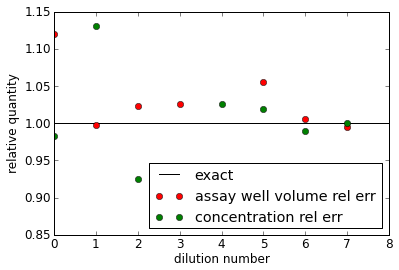In :
nreplicates = 1000
actual_volumes_n = numpy.zeros([nreplicates, ndilutions], numpy.float64)
actual_concentrations_n = numpy.zeros([nreplicates, ndilutions], numpy.float64)
for replicate in range(nreplicates):
[actual_volumes_replicate, actual_concentrations_replicate] = echo_assay_dispense(C0, mix_volume, backfill_volume, ideal_dispense_volumes)
actual_volumes_n[replicate,:] = actual_volumes_replicate
actual_concentrations_n[replicate,:] = actual_concentrations_replicate
volumes_cv = (actual_volumes_n / ideal_volumes).std(0)
concentrations_cv = (actual_concentrations_n / ideal_concentrations).std(0)
quantity_cv = ((actual_volumes_n * actual_concentrations_n) / (ideal_volumes * ideal_concentrations)).std(0)

# Plot CVs
clf()
x = numpy.arange(ndilutions)
plot(x, concentrations_cv*100, 'ro', x, volumes_cv*100, 'go', x, quantity_cv*100, 'bo');
xlabel('dilution number');
ylabel('CV (%)');
legend(['concentration', 'volume', 'quantity'], loc='lower left');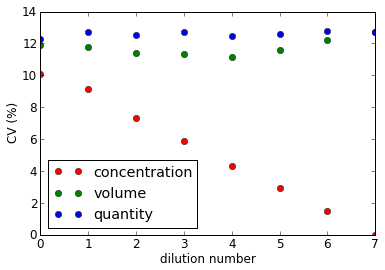How does a competitive inhibition look with the narrow dynamic range afforded by the LabCyte Echo?

In :
activity = numpy.zeros([ndilutions], numpy.float64)
for i in range(ndilutions):
activity[i] = competitive_inhibition(substrate_concentration, assay_compound_concentrations[i], enzyme_concentration, true_Ki, Km)

# Plot V0/Vmax for a single realization,
clf();
semilogx(ideal_concentrations, activity, 'ro');
xlabel('ideal [I] (M)');
ylabel('V0/Vmax');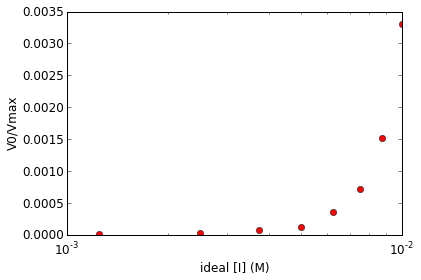V0 only goes up to a maximum of 0.012, but we can still see some curvature that could give a decent fit to Michaelis-Menten for an IC50. Let's try a number of replicates to see how much variation there is between measurements.

In :
activity = numpy.zeros([nreplicates,ndilutions], numpy.float64)
for replicate in range(nreplicates):
[assay_volumes, assay_compound_concentrations] = echo_assay_dispense(C0, mix_volume, backfill_volume, ideal_dispense_volumes)
for i in range(ndilutions):
activity[replicate,i] = competitive_inhibition(substrate_concentration, assay_compound_concentrations[i], enzyme_concentration, true_Ki, Km)

# Plot V0/Vmax for many measurements.
clf();
semilogx(ideal_concentrations, activity.transpose(), 'r-');
xlabel('ideal [I] (M)');
ylabel('V0/Vmax');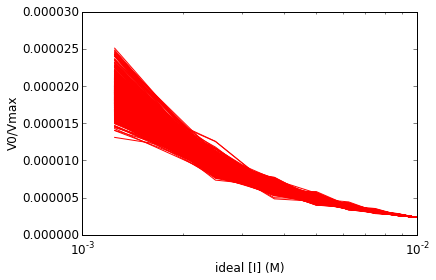Transforming this into IC50s, we see there is a fair amount of error.

In :
IC50s_echo = numpy.zeros([nreplicates], numpy.float64)
for replicate in range(nreplicates):
IC50s_echo[replicate] = fit_ic50(ideal_concentrations, activity[replicate,:])

clf()
nhist = 20
hist(numpy.log10(IC50s_echo), nhist);
xlabel('log IC50 (M)');
ylabel('P(IC50)');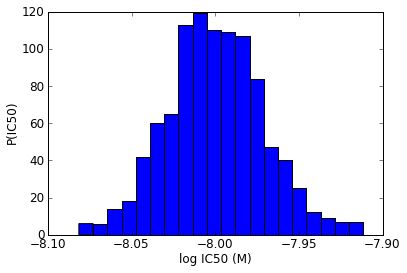Let's characterize the error for the Echo assay as a function of Ki.

In :
def echo_IC50s(true_Ki):
nreplicates = 1000
IC50s = numpy.zeros([nreplicates], numpy.float64)
for replicate in range(nreplicates):
[assay_volumes, assay_compound_concentrations] = echo_assay_dispense(C0, mix_volume, backfill_volume, ideal_dispense_volumes)
activities = numpy.zeros([ndilutions], numpy.float64)
for i in range(ndilutions):
activities[i] = competitive_inhibition(substrate_concentration, assay_compound_concentrations[i], enzyme_concentration, true_Ki, Km)
IC50s[replicate] = fit_ic50(ideal_concentrations, activities)
return IC50s

# Run simulation of many experiments.
pKis = numpy.array([-12, -11, -10, -9, -8, -7, -6, -5, -4, -3], numpy.float64);
Kis = 10**pKis
nKis = len(pKis)
echo_pIC50_bias = numpy.zeros([nKis], numpy.float64)
echo_pIC50_CV = numpy.zeros([nKis], numpy.float64)
for (i, Ki) in enumerate(Kis):
IC50s = echo_IC50s(Ki)
pIC50s = numpy.log10(IC50s)
pKi = pKis[i]
echo_pIC50_bias[i] = pIC50s.mean() - pKi;
echo_pIC50_CV[i] = pIC50s.std() / abs(pIC50s.mean())

In :
# Plot relative error in measured Ki values as a function of true Ki.
clf()
plot([pKis.min(), pKis.max()], [0, 0], 'k-', pKis, echo_pIC50_bias, 'ro');
xlabel('pKi');
ylabel('bias in measured pIC50');
axis([pKis.min(), pKis.max(), echo_pIC50_bias.min()-1, echo_pIC50_bias.max()+1]);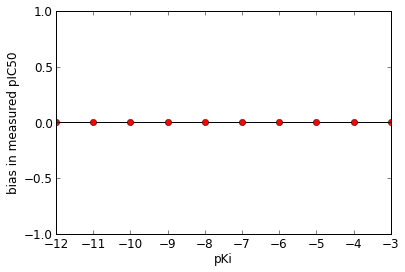In :
# Plot CV.
clf();
plot(pKis, echo_pIC50_CV*100, 'ro');
xlabel('pKi');
ylabel('measured pIC50 CV (%)');
axis([pKis.min(), pKis.max(), 0, 10]);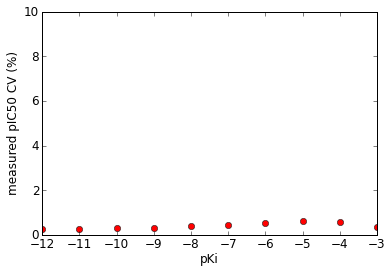## Comparison of Tecan Genesis and Labcyte Echo modeled performance¶

What do we get when we plot the Tecan Genesis and Echo results against each other?

In :
echo_pIC50_err = (echo_pIC50_CV*numpy.abs(pKis))
genesis_pIC50_err = (genesis_pIC50_CV*numpy.abs(pKis))

clf();
subplot(111, aspect='equal');
hold(True);
plot([pKis.min(), pKis.max()], [pKis.min(), pKis.max()], 'k-');
plot(pKis, pKis, 'ko');
errorbar(pKis + echo_pIC50_bias, pKis + genesis_pIC50_bias, xerr=2*echo_pIC50_err, yerr=2*genesis_pIC50_err, fmt='ro');
axis([pKis.min(), pKis.max(), pKis.min(), pKis.max()]);
xlabel('acoustic pIC50');
ylabel('tips pIC50');
title('95% confidence intervals shown');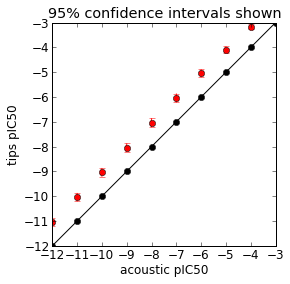In :
# data from Fig 1 of Fregau et al.
echo_IC50s = numpy.array([0.064, 0.486, 0.003, 0.002, 0.007, 0.003, 0.004, 0.052, 0.01362, 0.207, 0.158, 0.01164, 0.00633, 0.00358]) * 1e-6 # M
genesis_IC50s = numpy.array([0.817, 3.03, 0.146, 0.553, 0.973, 0.778, 0.445, 0.17, 0.112, 14.4, 0.25, 0.049, 0.087, 0.152]) * 1e-6 # M

echo_pIC50s = numpy.log10(echo_IC50s);
genesis_pIC50s = numpy.log10(genesis_IC50s);


Let's add the error bars we modeled to see if the variation is sufficient to bring the two sets of data into agreement.

In :
# Interpolate bias and CV for Echo and Genesis.
from scipy.interpolate import interp1d
echo_bias_interpolation = interp1d(pKis, echo_pIC50_bias);
echo_CV_interpolation = interp1d(pKis, echo_pIC50_CV);
genesis_bias_interpolation = interp1d(pKis, genesis_pIC50_bias);
genesis_CV_interpolation = interp1d(pKis, genesis_pIC50_CV);

# Compute error bars for Echo and Genesis.
echo_pIC50_err = (echo_CV_interpolation(echo_pIC50s)*numpy.abs(echo_pIC50s))
genesis_pIC50_err = (genesis_CV_interpolation(genesis_pIC50s)*numpy.abs(genesis_pIC50s))

# Plot with error bars.
figure();
subplot(111, aspect='equal');
hold(True);
plot([-9, -4], [-9, -4], 'k-');
errorbar(echo_pIC50s, genesis_pIC50s, xerr=2*echo_pIC50_err, yerr=2*genesis_pIC50_err, fmt='ro');
plot([-9, -6], [-9, -6], 'k-');
#axis('equal');
xlabel('pIC50 (acoustic)');
ylabel('pIC50 (tips)');
title('95% confidence intervals shown');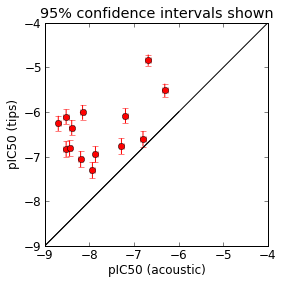What if we correct for the bias for each instrument?

In :
# Plot.
figure();
subplot(111, aspect='equal');
hold(True);
plot([-9, -4], [-9, -4], 'k-');
errorbar(echo_pIC50s, genesis_pIC50s, xerr=2*echo_pIC50_err, yerr=2*genesis_pIC50_err, fmt='ro');
errorbar(echo_pIC50s - echo_bias_interpolation(echo_pIC50s), genesis_pIC50s - genesis_bias_interpolation(genesis_pIC50s), xerr=2*echo_pIC50_err, yerr=2*genesis_pIC50_err, fmt='go');
plot([-9, -6], [-9, -6], 'k-');
#axis('equal');
xlabel('pIC50 (acoustic)');
ylabel('pIC50 (tips)');
legend(['ideal', 'original', 'bias corrected'], 'upper right');
title('95% confidence intervals shown');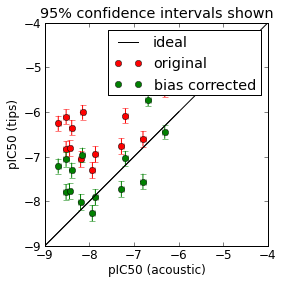The assays are in much better agreement at this point, but it does not appear that the specified imprecision of the Tecan Genesis and Labcyte Echo are sufficient to explain the remaining discrepancies.